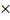# A massis moving with a constant velocity parallel to the-axis. Its angular momentum with respect to the origin a) Is zero b) Remains constant c) Goes on increasing d) Goes on decreasing

## Question ID - 100515 :- A massis moving with a constant velocity parallel to the-axis. Its angular momentum with respect to the origin a) Is zero b) Remains constant c) Goes on increasing d) Goes on decreasing

3537

(b)

Angular momentumMonmentumperpendicular distance of line of action of momentum w.r.t.

point of rotationThe quantities on the right side of the equation are not changing. Thus, magnitude is constant

Next Question :
 A trolley is moving horizontally with a velocity ofm/s w.r.t. earth. A man starts running in the direction of motion of trolley from one end of the trolley with a velocityw.r.t. the trolley. After reaching the opposite end, the man turns back and continues running with a velocity of 1.5m/s w.r.t. trolley in the backward direction. If the length of the trolley isthen the displacement of the man with respect to earth, measured as a function of time, will attain a maximum value of a)b)c)d)